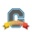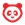# Community

cancel
Showing results for
Show  only  | Search instead for
Did you mean:New Member

## Weighting Assignments Within A Group

I want the assignment grades that are imported from MyLab Math into my Online Assignments group in Canvas to each be worth the same amount.  Even though some assignments are worth 9 points while others are worth 15 points, I want each assignment to be weighted the same within that assignment group. I have been able to set the weight for each group but am unsure about how to make sure that all assignments within a group are worth the same amount of points.

Tags (3)
1 SolutionCommunity Coach

Hi Jana,

Within an assignment group, assignments are weighted based on how many points they are worth.  You will need to adjust the points on the assignments themselves so that they are worth the same number of points in order for them to have the same weight within the assignment group.

Here are the basic instructions for editing points on an assignment:

Since the assignments are coming from MathLab, though, you may need to go to MathLab to adjust the points.

Mike

6 RepliesCommunity Coach

Hi Jana,

Within an assignment group, assignments are weighted based on how many points they are worth.  You will need to adjust the points on the assignments themselves so that they are worth the same number of points in order for them to have the same weight within the assignment group.

Here are the basic instructions for editing points on an assignment:

Since the assignments are coming from MathLab, though, you may need to go to MathLab to adjust the points.

MikeNew Member

Thanks. That’s what I did.

Respectfully,

J. Talley, PhD

Department of Mathematics and Statistical Sciences

Jackson State University

601-979-1740

jana.r.talley@jsums.eduCommunity Contributor

I am confused. Are we locked in with Canvas to only one method to weight assignments within a Category?

In our LMS from a decade ago, we could change the weightings on the individual items in a category. For example, with N assignments in a given category, we could do any one of these options:

* Total Score = (sum of all achieved points) / (sum of all possible points) <-- current approach in Canvas

* Total Score = (1/N) * sum of (percent score on assignment j) <-- all assignments equally weighted regardless of their points

* Total Score = sum of (weighting factor for assignment j) * (percent score on assignment j) <-- apply a weighting factor to each assignment

AFAIR, the first two changes were implemented with simple switches in the settings for the category, while the third option required a bit of programming skills.Community Coach

Hello Jeff,

Canvas does not have the some weighting options as some other LMSs such as Blackboard do.  The only way weights for assignments within a category are calculated is what was called "proportional weighting" in Blackboard.

MikeCommunity Contributor

Is Feature Request posted anywhere to ask that we be allowed to set variations in weighting factors within a Category?

--

JJWCommunity Team